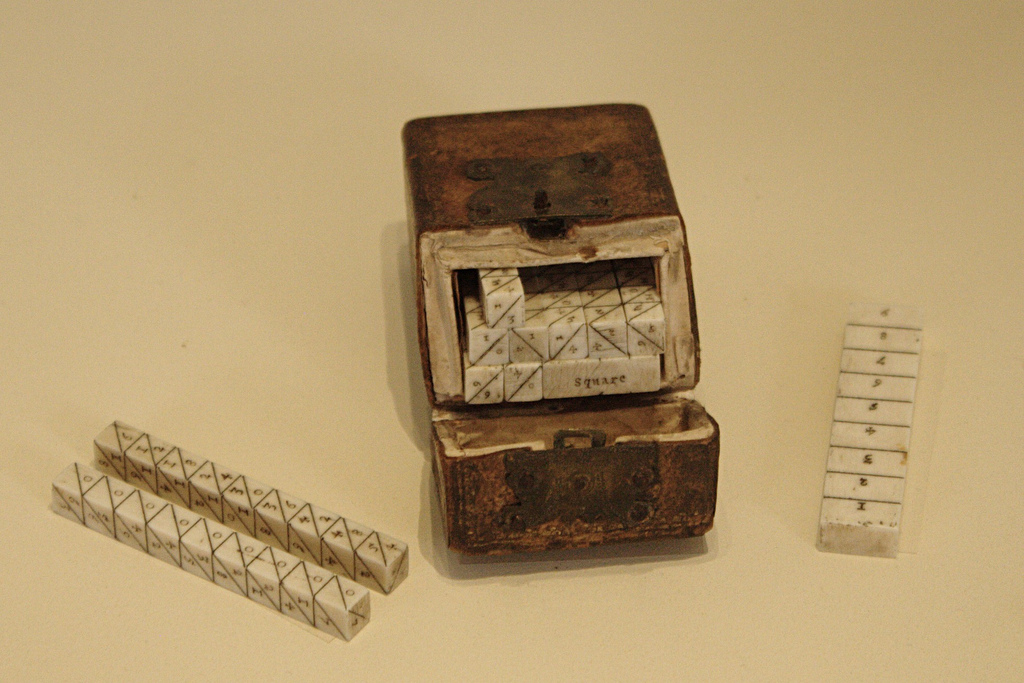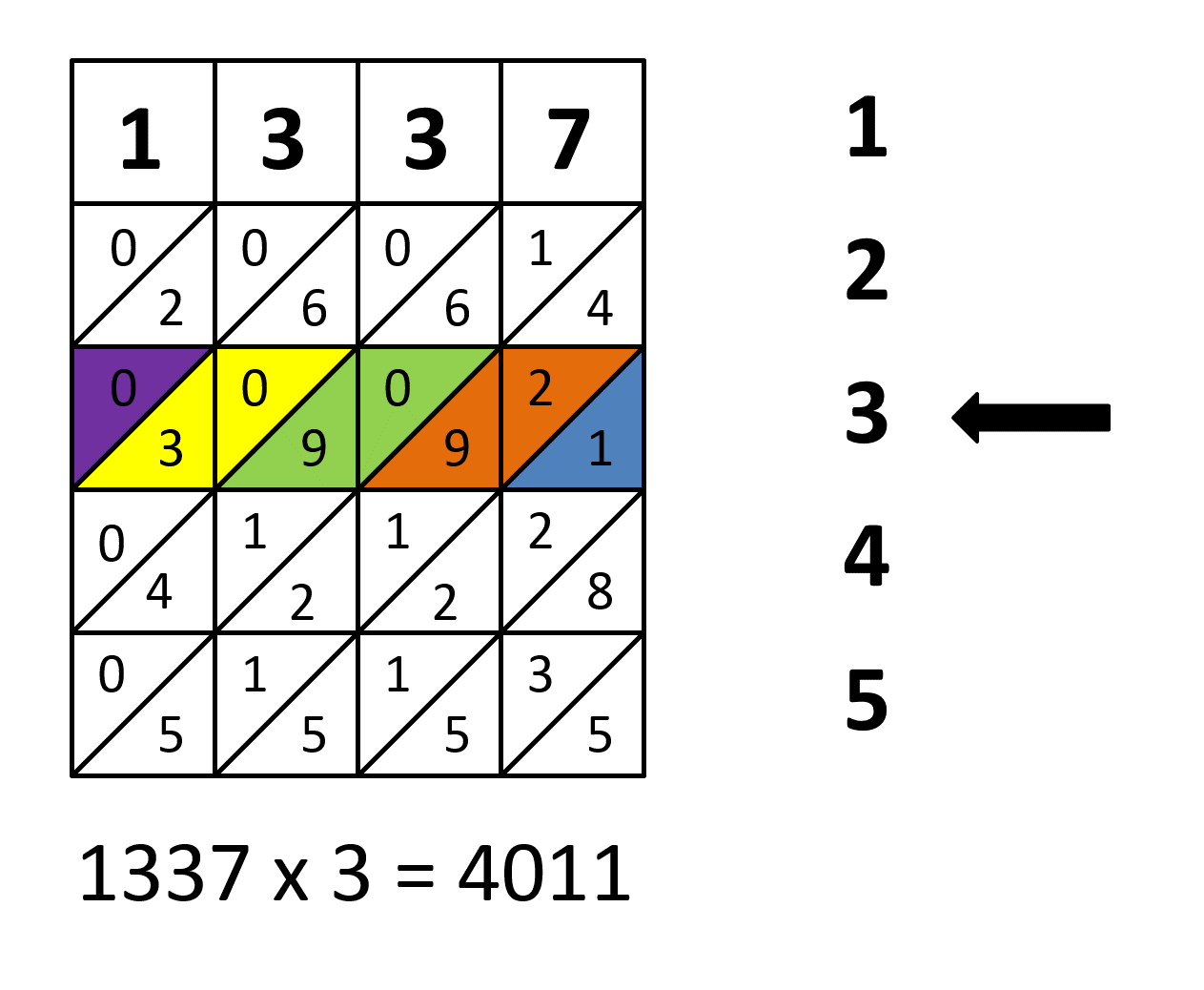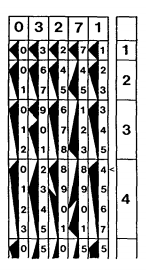# Bone Up On Your Multiplication SkillsJohn Napier was a Scottish physicist, mathematician, and astronomer who usually gets the credit for inventing logarithms. But his contributions to simplifying mathematics and building shorthand solutions didn’t end there. In the course of performing the many calculations he needed to practice these subjects in the 1500s, Napier invented a kind of computing mechanism for multiplication. It’s a physical manifestation of an old system known as lattice multiplication or gelosia.

Lattice multiplication makes use of the multiplication table in order to multiply huge numbers together quickly and easily. It is thought to have originated in India and moved west into Europe. When the lattice method reached Italy, the Italians named it gelosia after the trellised window covering it resembled, which was commonly used to keep prying eyes away from one’s possessions and wife.

### A Numerical Kaleidoscope

If you’ve never heard of lattice multiplication, let me briefly explain how it works using a couple of two-digit numbers. Say you want to multiply 12 by 34. You would still have to break it down into smaller multiplication problems and add these partial products to find the answer. The lattice method makes the process faster and more visually interesting. I think it reveals the beauty of numbers.The use of lattice multiplication to solve for 12 x 34.

The multiplicands are set up perpendicularly with one digit written horizontally and the other written vertically down the right side. Then, a framework of boxes is drawn to the scale of the two numbers being multiplied. Each box must be divided diagonally to separate the tens from the ones in the intermediate multiplication problems. If the intermediate value is less than 10, a zero is written in the tens column.

After the lattice has been filled out, the values delineated by the diagonals are added together. This is done from right to left, starting from the ones column represented with here with a blue parallelogram and moving left.

So, working from right to left and top to bottom: three times two is six. Three times one is three. Repeat the process using the four from the ones column of ’34’: four times two is eight, and four times one is four. From there, it’s just a matter of simple addition performed diagonally and from right to left.

The first value, eight, is by itself. Therefore the ones value of 12 x 34 is eight. Moving left to the tens column, we add 6, 0, and 4 to get 10. The 1 from ’10’ is carried to the next diagonal, so ignoring the zeroes, three plus the carried one equals four. Thus, 12 x 34 = 408.An ivory set of Napier’s Bones from around 1650. Image via Marc Kjerland.

### It’s a Numbers Game

John Napier had a great deal of calculating to do. The lattice multiplication method was a big help, but he had so much to do that drawing out a lattice for every equation was cumbersome. He needed a sturdy and reusable implementation so he built a set of thin rods that included all possible permutations of the gelosia method.

He made one rod for each number from 0-9. The rods were each divided vertically into nine segments and the sequential products written in these squares. All that needed to be done to use them was to arrange the rods to represent one of the multiplicands and line up the row-numbering marker next to them.The use of lattice multiplication to solve for 1337 x 3.

Adding the diagonals from right to left: in the ones place of the answer is 1. Two plus nine is 11, and the one is carried to the green diagonal to bring its total to 10. The one from that result is carried to the yellow diagonal, which brings its total to 4 and the answer to 4011.

To multiply 1337 by a larger number like 34, add the diagonals of row 3 and row 4 together and do the necessary carries. These rods can also be used for division, roots, and cube root calculations.

Napier wrote a treatise called Rabdologiæ that describes the rods and their use and discusses two other analog calculating devices that were not quite as popular. Rabdologiæ is a term he coined from the Greek rabdos meaning ‘rod’ and logos meaning ‘computation’ or ‘reckoning’. Napier’s Bones caught on fairly quickly and were made from many different materials. Nicer sets would be carved from bone or ivory. They were popular and useful enough to spark the imaginations of others into improving upon them.

### Everybody’s a CriticThe use of Genaille-Lucas rulers to solve 3271 x 4. Image via William Aspray, Computing Before Computers.

A German mathematician named Gaspard Schott tried to address such issues as containing the bones and lining them up adequately for performing calculations. His solution involved several cylinders with complete sets of Napier’s Bones on each one. All of these cylinders were contained in a box with slits on the top for reading off the numbers. This was a novel idea, but in practice, the numbers were spaced too far apart which defeats the purpose of a having calculating aid.

Henri Genaille, a French civil engineer with several arithmetic aids to his name created an alternate version of Napier’s Bones by complete accident. He was attempting to solve a problem given by Tower of Hanoi inventor Édouard Lucas at an academic meeting. He called his version Genaille-Lucas rulers. They work a lot like Napier’s Bones, but they don’t require any calculations—the arrows trace a path from left to right that reveals the answer.

### Mechanical Progress

The quest for a mechanical calculating device certainly didn’t begin or end with Gaspard Schott’s box of spinning cylinders. The abacus is probably the oldest general purpose calculator known to man. In the 1640s, Pascal created a device to make tax calculation easier. An Austrian engineer named Curt Herzstark built the CURTA mechanical calculator, a small handheld device that could perform the four basic mathematical functions plus square root calculations. Not only did it save him time, it saved him from a concentration camp.

## 5 thoughts on “Bone Up On Your Multiplication Skills”

1. DV82XL says:

Sir Walter Scott in his story, Fortunes of Niqel, makes one of the characters, Davie Ramsay swear by “ the bones of the immortal Napier,” which I always thought was as good a math-base oath as one could imagine.

2. Those Genaille-Lucas rulers are pretty neat. Might be useful on small microcontrollers for dealing with numbers bigger than standard library functions will let them handle, without resorting to floating point? When I saw them, I had a sudden idea that they could be implemented with some arrays, and pointer arithmetic? But I haven’t had time yet to actually think about it enough to see if that’s a viable approach.

1. arachnidster says:

Interesting idea, but I suspect they end up less efficient than standard methods of implementing long binary multiplication.

3. Ren says:

Nice article! Thanks!

4. Stephen says:

Napier also invented an “abacus” using binary notation on a chessboard! This could be used to add, subtract, multiply, divide, and extract square roots.

Please be kind and respectful to help make the comments section excellent. (Comment Policy)

This site uses Akismet to reduce spam. Learn how your comment data is processed.